Courses

# Stability - MCQ Test

## 15 Questions MCQ Test GATE Electrical Engineering (EE) 2022 Mock Test Series | Stability - MCQ Test

Description
This mock test of Stability - MCQ Test for Electronics and Communication Engineering (ECE) helps you for every Electronics and Communication Engineering (ECE) entrance exam. This contains 15 Multiple Choice Questions for Electronics and Communication Engineering (ECE) Stability - MCQ Test (mcq) to study with solutions a complete question bank. The solved questions answers in this Stability - MCQ Test quiz give you a good mix of easy questions and tough questions. Electronics and Communication Engineering (ECE) students definitely take this Stability - MCQ Test exercise for a better result in the exam. You can find other Stability - MCQ Test extra questions, long questions & short questions for Electronics and Communication Engineering (ECE) on EduRev as well by searching above.
QUESTION: 1

### The forward-path transfer function of a ufb system is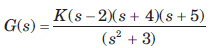For system to be stable, the range of K is

Solution: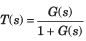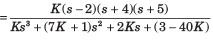Routh table is as shown in fig. S.6.211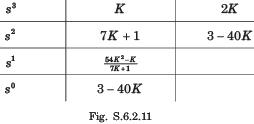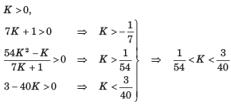QUESTION: 2

Solution:
QUESTION: 3

### The open-loop transfer function of a ufb system is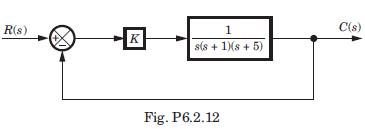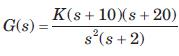The closed loop system will be stable if the value of K is

Solution: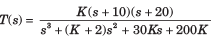Routh table is as shown in fig.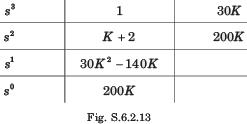200K > 0 → K > 0, 30K2 - 140K > 0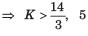satisfy this condition.

QUESTION: 4

The closed loop transfer function for this system is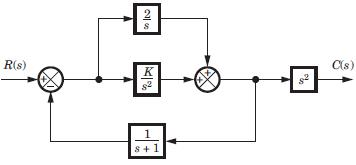Solution:

First combine the parallel loop K/s2
and 2/s giving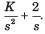Then apply feedback formula with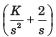and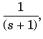and then multiply with s2.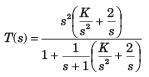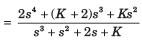QUESTION: 5

The poles location for this system is shown in fig.The value of K is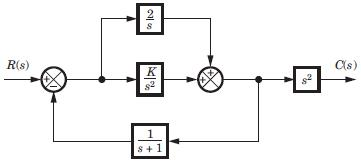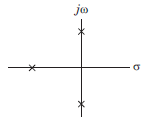Solution:

Denominator = s3 + s2 + 2s + K Routh table is as shown in fig.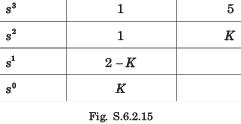Row of zeros when K = 2,
s2 + 2 = 0, ⇒ s = -1, j√2, - j√2

QUESTION: 6

The forward path transfer of ufb system is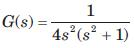The system is

Solution:
QUESTION: 7

The forward-path transfer function of a ufb system is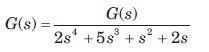The system is

Solution:

Closed loop transfer function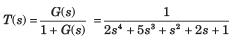Routh table is as shown in fig. S.6.2.28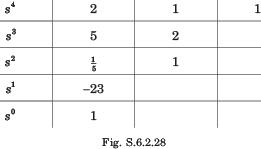2 RHP poles so unstable.

QUESTION: 8

The open loop transfer function of a system is as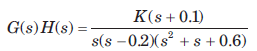The range of K for stable system will be

Solution:

The characteristic equation is 1 + G(s)H(s) = 0

⇒ s(s - 0.2)(s2 + s + 0.6)+K(s + 0.1) = 0
s4 +0.8 s3 +0.4s2 +(K - 0.12)s +0.1K = 0
Routh table is as shown in fig. S.62.29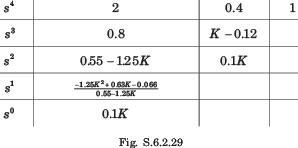K > 0, 055 -125K > 0 ⇒ K < 0.44 -125K2 +0.63K -0066 >0
(K - 0.149)(K - 0355) < 0, 0.149 < K < 0.355

QUESTION: 9

The open-loop transfer function of a ufb control system is given by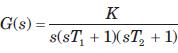For the system to be stable the range of K is

Solution:

Characteristic equation

s(sT1 + 1)(sT2 +1) + K = 0
T1T2s3 + (T1 + T2)s2 + s + K = 0
Routh table is as shown in fig S.6.2.30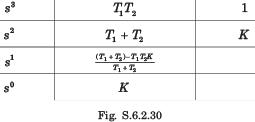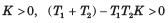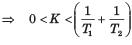QUESTION: 10

If the roots of the have negative real parts, then the response is ____________

Solution:

If the roots of the have negative real parts then the response is bounded and eventually decreases to zero.

QUESTION: 11

The closed loop transfer function of a system is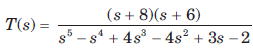The number of poles in RHP and in LHP are

Solution: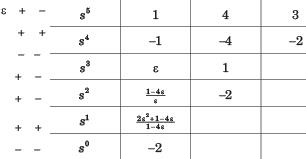3 RHP, 2 LHP poles.

QUESTION: 12

The closed loop transfer function of a system is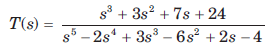The number of poles in LHP, in RHP, and on jω - axis are

Solution: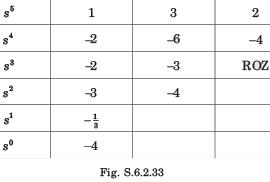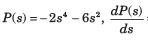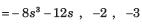No sign change exist from the s4 row down to the s0 row.
Thus, the even polynomial does not have RHP poles. Therefore because of symmetry all four poles must be on jw -axis.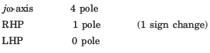QUESTION: 13

For the system shown in fig. the number of poles on RHP, LHP, and imaginary axis are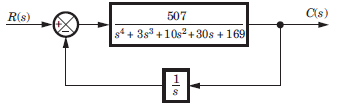Solution:

Closed loop transfer function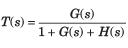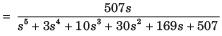Routh table is as shown in fig. S.6.2.34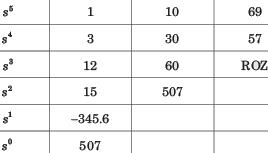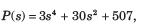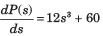From s4 row down to s0 there is one sign change. So LHP–1 + 1= 2 pole. RHP–1 pole, jw - axis - 2 pole.

QUESTION: 14

If a system is given unbounded input then the system is:

Solution:

If the system is given with the unbounded input then nothing can be clarified for the stability of the system.

QUESTION: 15

For the open loop system of fig. location of poles on RHP, LHP, and an jω - axis are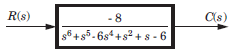Solution:

Routh table is as shown in fig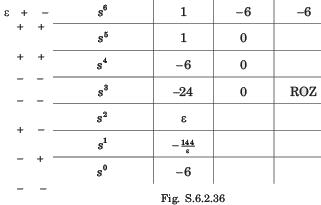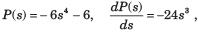Them is two sign change from the s4 mw down to the s° row. So two roots are on RHS. Because of symmetry rest two roots must be in LHP. From s6 to s4 there is 1 sign change so 1 on RHP and 1 on LHP.
Total LHP 3 root, RHP 3 root.